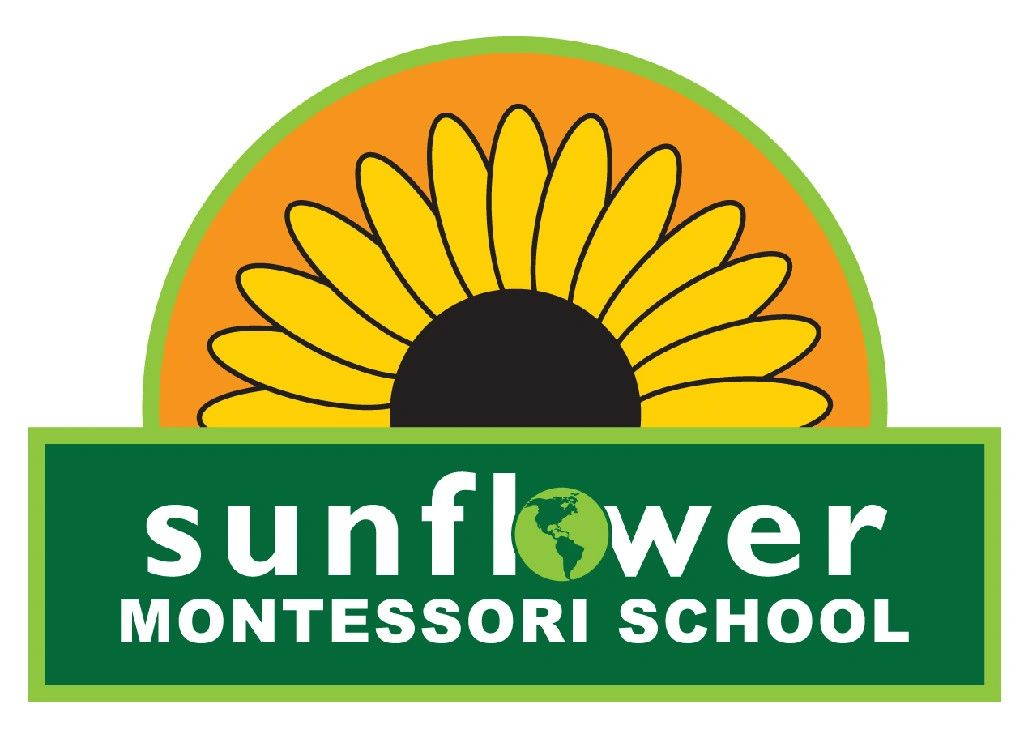Quality Infant, Toddler and
Primary CareSunflower Montessori School - The Primary Classroom Environment: Math

Math is perhaps one of the most abstract concepts that the human mind has encountered. The ability to count, compute, and use numerical relationships are among the most significant among human achievements. The number system, which has been created over thousands of years, is an abstract invention. It began with the realization of one and then more than one. It is marvelous to see the readiness of the child’s understanding of this same concept.

In the Montessori classroom, there are several “families” of Math presented to the child: arithmetic, geometry, statistics and calculus. The concepts covered in the Primary class are: numeration, the decimal system, computation, the arithmetic tables, whole numbers, fractions, and positive numbers. We offer arithmetic to the child from age four to age five and six.

Arithmetic is the science of computing positive ‘real’ numbers. It is specifically the process of addition, subtraction, multiplication and division. Additionally the materials of the Primary Montessori classroom present sensorial experiences in geometry and algebra.

The Montessori materials help the child construct precise order. In the class, the child is offered material and experiences to help him build internal order.

The mathematical material gives the child his own mathematical experience and to arrive at individual work. There are some teacher directed activities but these are followed with activities for the individual.

The Exercises in arithmetic are grouped. There is some sequential work and some parallel work. The first group is Numbers through Ten. The experiences in this group are sequential. When the child has a full understanding of numbers through ten, the second group,

Then the Decimal System can be introduced. The focus here is on the hierarchy of the decimal system and how the system functions. It also starts the child on the Exercises of simple computations, which are the operations of arithmetic.

When the child is ready for math, the absorption is as easy and natural as for other areas of knowledge. It is empowering and brings the child to a level of confidence and joy in another path of culture.

 Read More Introduction Practical Life Sensorial Language Writing Reading Math Geography Science Art and Music